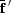disable zoom     view article Figure 11 Eigenvalues of the average resonant scattering tensors at the Se K edge. The plot represents the spectra of the three eigenvalues of thetensor (upper half) and the three eigenvalues of thetensor (lower half) as a function of X-ray energy at the Se K edge. The red curves represent the eigenvalues corresponding to the y-axis direction (i.e. the crystal b axis). The black and green curves represent eigenvalues corresponding to two mutually orthogonal directions in the xz plane which vary with X-ray energy [i.e. there is some orientational dispersion (Lippmann et al., 1998) in this plane].JOURNAL OFAPPLIEDCRYSTALLOGRAPHY
ISSN: 1600-5767
Volume 38| Part 1| January 2005| Pages 168-182